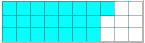# Understanding Percent

Percent concepts are introduced in this unit. Conversions between percents, fractions and decimals are also covered. Try our sample lessons below, or browse other units of instruction.Understanding Percent Description Meaning of Percent To define and understand percent. To write ratios as fractions, decimals and percentages. Writing Fractions as Percents To write a fraction as a percentage by converting it to an equivalent fraction with a denominator of 100. To write a fraction as a percentage by dividing its numerator by its denominator, then converting the resulting decimal to a percentage. Writing Decimals as Percents To convert a decimal to percentage by moving the decimal point two places to the right. Decimals with varying place values are presented. Connections to money are made. Writing Percents as Decimals To convert a percent to a decimal by moving the decimal point two places to the left. Both whole-number percentages and decimal percentages are presented. Writing Percents as Fractions To write a percent as a fraction in lowest terms. The GCF method is used to reduce fractions. Both whole-number and decimal percents are presented. Percents Less Than 1 or Greater Than 100 To understand the value of percentages greater than 100 and percentages less than 1. To write these special percentages as decimals or as fractions in lowest terms. To write decimals as a percentage greater than 100 or less than 1. Practice Exercises To complete 10 additional exercises as practice. To assess students' understanding of all percentage concepts learned. Challenge Exercises To solve 9 additional problems that challenge students' understanding of all concepts in this unit. Students are asked to convert between fractions, decimals and percents by finding the missing value. Problems are presented in a game format. Solutions To review complete solutions to all exercises presented in this unit. Includes the problem, step-by-step solutions, final answer and units for each exercise. Learning Objectives Learn about writing fractions as percents and decimals as percents, writing percents as decimals and percents as fractions, percents less than 1 or greater than 100.